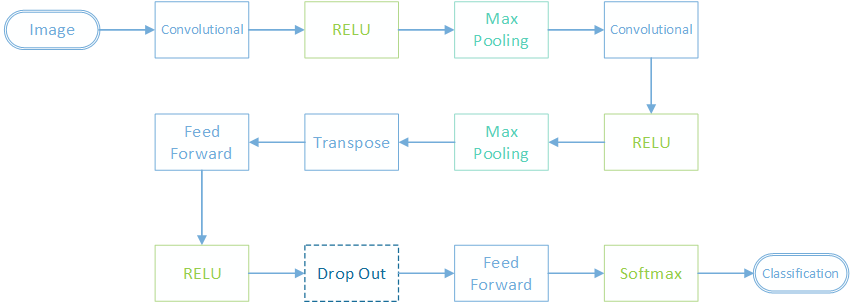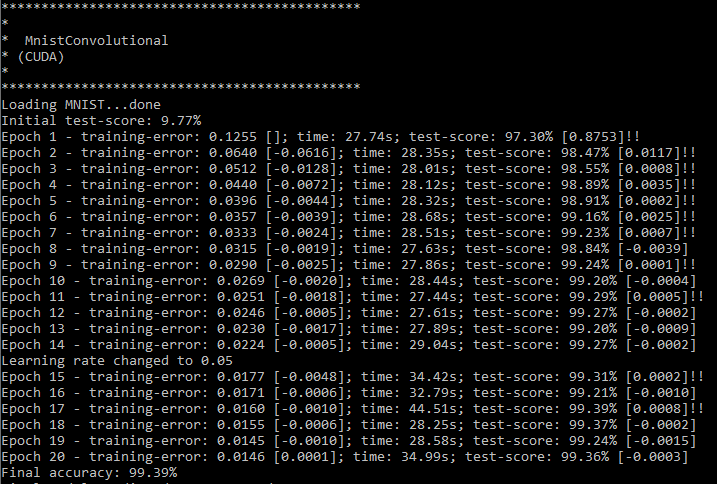# Learning to recognise handwritten digits (MNIST) with convolutional neural networks gives a higher classification accuracy (and a longer training time)

## Motivation

Originally designed to mimic the neurons in the visual cortex, convolutional neural networks (CNN) are particularly good at classifying images. They work by sliding a filter around the input image, and multiplying the image by this filter at each step to create a new "filtered" image.

A layer in a CNN might have multiple filters, so there might be multiple output images, each multiplied by a different filter. The CNN learns to improve each of the filter weights by backpropagation.

Eventually the output images are flattened into a single vector and feed forward layer performs the actual classification - "car" or "cat" etc.

CNN generalise well when they are stacked on top of each other. Lower level filters tend to detect things like edges, and upper level filters use those lower level features as part of their own higher level feature detection.

Recently, CNN have also been shown to work well for natural language processing tasks - the filter slides down a sentence capturing n words at a time and translating that word group into a feature in the same way that edges are recognised in images.

Previously we tried to classify images of handwritten digits in the MNIST data set with a feed forward network and got an accuracy above 98%. In this tutorial we will use a convolutional neural network to get an accuracy above 99%.

## Network Design

This is the first tutorial that really uses "deep learning" - stacking neural network layers that are more than a few layers deep. In this case, the network is six layers deep (not counting activation and drop out layers).The first layer is a convolutional layer, followed by a RELU activation.

This is followed by a max pooling layer. Max pooling is like a convolutional layer, but rather than sliding a filter around and multiplying the input images by the filter weights, max pooling layers take the highest pixel value in each filter and passes it to the next layer. This has the effect of shrinking each input image, usually by a factor of 4 - as a (2, 2) max pool filter is the most commonly used max pooling filter size.

After the max pooling there is another sequence of convolutional, RELU and max pooling layers.

At this point the data is transposed. In Bright Wire the convolutional layers operate on data laid out in columns but the other layers expect training examples to be stored in rows. A matrix transpose layer converts between the two sets of expectations.

Finally two feed forward layers (separated by more RELU and dropout layers) make the actual classification.

Bright Wire includes a helper class to load images in the MNIST data set. As before, MNIST contains images of hand written digits along with the classification label corresponding to what the handwritten digit actually is. So a picture of a "3" will have an output classification label of 3.

These can be one hot encoded so that each output classification is a ten digit vector which is set to zero except for the index of the classification label which is set to 1.

The expected data table format is a 3D tensor column with the image data (a 3D tensor is a list of matrices, one for each input image channel (red, green blue) or in this case since MNIST is black and white, a list with a single matrix for the black and white pixel values) followed by the output vector column. The output vector in this case contains the one hot encoded classification labels (0, 1, 2, 3 etc).

```public static IRowOrientedDataTable Build3DTensorToVectorDataTable(IBrightDataContext context, Image[] images)
{
// create a 3D tensor => vector mapping
var dataTable = context.Create3DTensorToVectorTableBuilder();

foreach (var image in images) {
var (tensor, label) = image.AsFloatTensor(context);
}

return dataTable.BuildRowOriented();
}```

This function is invoked with the following code. Note that we're using the GPU linear algebra provider to train this network.

```// one hot encoding uses the index of the output vector's maximum value as the classification label
var errorMetric = graph.ErrorMetric.OneHotEncoding;

// configure the network properties
graph.CurrentPropertySet
.Use(graph.GaussianWeightInitialisation(false, 0.1f, GaussianVarianceCalibration.SquareRoot2N))
;```

The network is trained with Adam gradient descent and Gaussian weight initialisation for 20 epochs. The training rate is decreased at epoch 15 to help improve the final accuracy.

The first convolutional layer uses sixteen filters of size (5,5). This is the size of the input box that slides around the image. To preserve the image dimensions the image is zero padded. With padding, the output image will be the same size as the input image.

The second convolutional layer uses the same filter size of (5,5) but this time uses thirty two filters.

Each of the max pooling layers use a filter size of (2,2) but a stride of 2. The stride is the step size in which the box moves around the image. In this case the box of four pixels will measure every pixel once in the image without overlap and only one in four of the input pixels will be preserved in the output image. The other pixels will simply be dropped from consideration.

```graph.Connect(engine)
.AddConvolutional(filterCount: 16, padding: 2, filterWidth: 5, filterHeight: 5, xStride: 1, yStride: 1, shouldBackpropagate: false)
.AddMaxPooling(filterWidth: 2, filterHeight: 2, xStride: 2, yStride: 2)
.AddConvolutional(filterCount: 32, padding: 2, filterWidth: 5, filterHeight: 5, xStride: 1, yStride: 1)
.AddMaxPooling(filterWidth: 2, filterHeight: 2, xStride: 2, yStride: 2)
.Transpose()